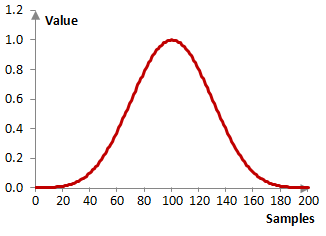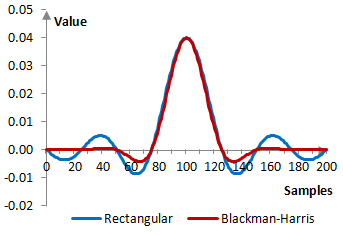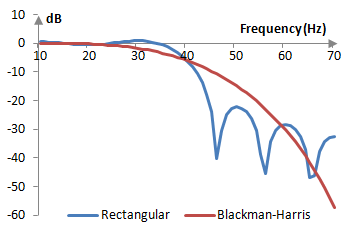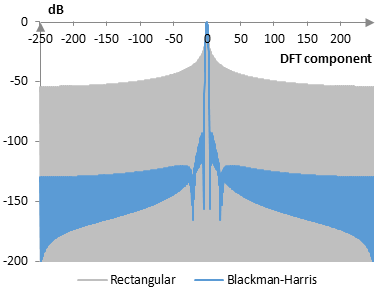# Blackman-Harris window

The Blackman-Harris window coefficients are given by the following formula

$$a(k)=0.35875-0.48829 \, \cos(\frac{2\pi k}{N-1})+0.14128 \, \cos(\frac{4\pi k}{N-1})$$ $$-0.01168 \, \cos(\frac{6\pi k}{N-1})$$

where N is the length of the filter and k = 0, 1, …, N – 1.

The Blackman-Harris window is a generalized cosine window (see Hamming window).

## An example Blackman-Harris window

Consider a finite impulse response (FIR) low pass filter of length N = 201. The following is the Blackman-Harris window.Given a sampling frequency of 2000 Hz and a filter cutoff frequency of 40 Hz, the impulse response of the filter with a rectangular window (with no window) and with the Blackman-Harris window is as follows.The magnitude response of the same filter is shown on the graph below.## Measures for the Blackman-Harris window

The following graph compares the discrete Fourier transform of the Blackman-Harris window and the rectangular window.The Blackman-Harris window measures are as follows.

 Coherent gain 0.36 Equivalent noise bandwidth 2.01 Processing gain -3.03 dB Scalloping loss -0.82 dB Worst case processing loss -3.85 dB Highest sidelobe level -92.0 dB Sidelobe falloff -14.4 dB / octave, -48.0 dB / decade Main lobe is -3 dB 1.90 bins Main lobe is -6 dB 2.66 bins Overlap correlation at 50% overlap 0.037 Amplitude flatness at 50% overlap 0.435 Overlap correlation at 75% overlap 0.459 Amplitude flatness at 75% overlap 1.000

Window

### Filtered HTML

• Freelinking helps you easily create HTML links. Links take the form of [[indicator:target|Title]]. By default (no indicator): Click to view a local node.